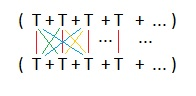# Series – some general rules

Up a level : Power Series
Previous page : Radius of convergence - another example
Next page : Some interesting examples based on the Fibonacci seriesLet us say we have two functions f(x) and g(x) that we know the Taylor expansion of.

• The series expansion of f(x)+g(x) equals the series expansions added.

This is because of the rules of the derivatives. It is not too hard to prove.

• The series expansion of f(x)g(x) equals the series expansions multiplied term by term. Collect all term by equal power.

The product rule derivatives of higher orders is${\left( {f \cdot g} \right)^{(n)}} = \sum\limits_{r = 1}^n {\left( {\begin{array}{*{20}{c}} n \\ r \end{array}} \right){f^{(r)}}{g^{(n - r)}}}$

I leave it to you to show that. From that it is not all too hard to show the above. In particular, the series expansion of a polynomial times a function can be found by multiplying the polynomial term by term with the series expansion of the function.

• The series expansion of f(x)/g(x) equals the series expansions divided by each other. Do long division with the terms “backwards” from the smallest to the highest power. This can be useful if you need just a few terms.
• The series expansion of f(g(x)) can be done by substituting in the expansion for g(x) into the expansion of f(x) and then expand what we get. Then we collect terms of equal powers in x. This method can be useful if we only need a few term, or if one of the functions is a finite polynomial. We have, for example,$\begin{gathered} \sin ({x^2}) = ({x^2}) - \frac{{{{({x^2})}^3}}}{{3!}} + \frac{{{{({x^2})}^5}}}{{5!}} - \frac{{{{({x^2})}^7}}}{{7!}} + ... \hfill \\ \quad \quad \quad = {x^2} - \frac{{{x^6}}}{{3!}} + \frac{{{x^{10}}}}{{5!}} - \frac{{{x^{14}}}}{{7!}} + ... \hfill \\ \end{gathered}$

and$\begin{gathered} {\sin ^2}x = {(\sin x)^2} = {\left( {x - \frac{{{x^3}}}{{3!}} + \frac{{{x^5}}}{{5!}} - \frac{{{x^7}}}{{7!}} + ...} \right)^2} \hfill \\ \quad \quad \quad = {x^2} - 2 \cdot \frac{{{x^4}}}{{3!}} + 2 \cdot \frac{{{x^6}}}{{5!}} - 2 \cdot \frac{{{x^8}}}{{7!}} + ... \hfill \\ \quad \quad \quad \;\quad \;\quad \quad + \frac{{{x^6}}}{{{{(3!)}^2}}} - 2 \cdot \frac{{{x^8}}}{{3!5!}} + ... \hfill \\ \quad \quad \quad \;\quad \;\quad \quad ... \hfill \\ \quad \quad \quad = {x^2} - \frac{{{x^4}}}{3} + \left( {\frac{2}{{5!}} + \frac{1}{{{{(3!)}^2}}}} \right){x^6} - \left( {\frac{2}{{7!}} + \frac{2}{{3!5!}}} \right){x^8} + ... \hfill \\ \quad \quad \quad = {x^2} - \frac{{{x^4}}}{3} + \frac{2}{{45}}{x^6} - \frac{1}{{315}}{x^8} + ... \hfill \\ \end{gathered}$

To get the later one, remember that have to multiply across two bracket, and that all but those that are squared will be two of.• The series expansion of f(-x) is the series expansion with the sign of every odd term changed.Up a level : Power Series
Previous page : Radius of convergence - another example
Next page : Some interesting examples based on the Fibonacci seriesLast modified: Mar 7, 2019 @ 20:41